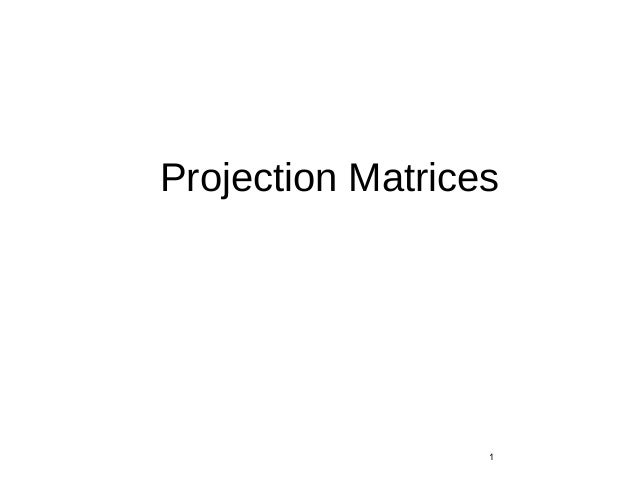Successfully reported this slideshow.Upcoming SlideShare
×

# Projection Matrices

999 views

Published on

A computer monitor is a 2D surface. A 3D scene rendered by OpenGL must be projected onto the computer screen as a 2D image. GL_PROJECTION matrix is used for this projection transformation.

Published in: Engineering
• Full Name
Comment goes here.

Are you sure you want to Yes No• Be the first to comment

### Projection Matrices

1. 1. Projection Matrices 1
2. 2. Objectives • Derive the projection matrices used for standard OpenGL projections • Introduce oblique projections • Introduce projection normalization 2
3. 3. Normalization • Rather than derive a different projection matrix for each type of projection, we can convert all projections to orthogonal projections with the default view volume • This strategy allows us to use standard transformations in the pipeline and makes for efficient clipping 3
4. 4. Pipeline View 4 modelview transformation projection transformation perspective division clipping projection nonsingular 4D → 3D against default cube 3D → 2D
5. 5. Notes • We stay in four-dimensional homogeneous coordinates through both the modelview and projection transformations • Both these transformations are nonsingular • Default to identity matrices (orthogonal view) • Normalization lets us clip against simple cube regardless of type of projection • Delay final projection until end • Important for hidden-surface removal to retain depth information as long as possible 5
6. 6. Orthogonal Normalization glOrtho(left,right,bottom,top,near,far) 6 normalization ⇒ find transformation to convert specified clipping volume to default
7. 7. Orthogonal Matrix • Two steps • Move center to origin T(-(left+right)/2, -(bottom+top)/2,(near+far)/2)) • Scale to have sides of length 2 S(2/(left-right),2/(top-bottom),2/(near-far)) 7                     − + − − + − − − − − − 1000 2 00 0 2 0 00 2 nearfar nearfar farnear bottomtop bottomtop bottomtop leftright leftright leftright P = ST =
8. 8. Final Projection •Set z =0 •Equivalent to the homogeneous coordinate transformation •Hence, general orthogonal projection in 4D is 8             1000 0000 0010 0001 Morth = P = MorthST
9. 9. Oblique Projections •The OpenGL projection functions cannot produce general parallel projections such as •However if we look at the example of the cube it appears that the cube has been sheared •Oblique Projection = Shear + Orthogonal Projection 9
10. 10. General Shear side view 10 top view
11. 11. Shear Matrix xy shear (z values unchanged) Projection matrix General case: 11             − − 1000 0100 0φcot10 0θcot01 H(θ,φ) = P = Morth H(θ,φ) P = Morth STH(θ,φ)
12. 12. Equivalency 12
13. 13. Effect on Clipping • The projection matrix P = STH transforms the original clipping volume to the default clipping volume 13 top view DOP DOP near plane far plane object clipping volume z = -1 z = 1 x = -1 x = 1 distorted object (projects correctly)
14. 14. Simple Perspective Consider a simple perspective with the COP at the origin, the near clipping plane at z = -1, and a 90 degree field of view determined by the planes x = ±z, y = ±z 14
15. 15. Perspective Matrices Simple projection matrix in homogeneous coordinates Note that this matrix is independent of the far clipping plane 15             − 0100 0100 0010 0001 M =
16. 16. Generalization N = 16             − 0100 βα00 0010 0001 after perspective division, the point (x, y, z, 1) goes to x’’ = x/z y’’ = y/z Z’’ = -(α+β/z) which projects orthogonally to the desired point regardless of α and β
17. 17. Picking α and β 17 If we pick α = β = nearfar farnear − + farnear farnear2 − ∗ the near plane is mapped to z = -1 the far plane is mapped to z =1 and the sides are mapped to x = ± 1, y = ± 1 Hence the new clipping volume is the default clipping volume
18. 18. Normalization Transformation original clipping volume 18 original object new clipping volume distorted object projects correctly
19. 19. Normalization and Hidden- Surface Removal •Although our selection of the form of the perspective matrices may appear somewhat arbitrary, it was chosen so that if z1 > z2 in the original clipping volume then the for the transformed points z1’ > z2’ •Thus hidden surface removal works if we first apply the normalization transformation •However, the formula z’’ = -(α+β/z) implies that the distances are distorted by the normalization which can cause numerical problems especially if the near distance is small 19
20. 20. OpenGL Perspective •glFrustum allows for an unsymmetric viewing frustum (although gluPerspective does not) 20
21. 21. OpenGL Perspective Matrix • The normalization in glFrustum requires an initial shear to form a right viewing pyramid, followed by a scaling to get the normalized perspective volume. Finally, the perspective matrix results in needing only a final orthogonal transformation 21 P = NSH our previously defined perspective matrix shear and scale
22. 22. Why do we do it this way? • Normalization allows for a single pipeline for both perspective and orthogonal viewing • We stay in four dimensional homogeneous coordinates as long as possible to retain three-dimensional information needed for hidden- surface removal and shading • We simplify clipping 22